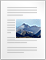# Complex Numbers

[latexpage]

Complex numbers are the numbers of the form a+ib where $i=\sqrt{(-1)}$ and a and b are real numbers.

Definition:- Complex numbers are defined as an ordered pair of real numbers like (x,y) where

$z=(x,y)=x+iy$

and both x and y are real numbers and x is known as real part of complex number and y is known as imaginary part of the complex number.

Let z1=x1+iy1 and z2=x2+iy2 then

z1+z2=(x1+x2)+i(y1+y2)

Subtraction

z1-z2=(x1-x2)+i(y1-y2)

Multiplication

(z1.z2)=(x1+iy1).(x2+iy2)

Division

To divide complex number by another , first write quotient as a fraction. Then reduce the fraction to rectangular form by multiplying the numerator and denominator by the complex conjugate of the denominatormaking the denominator real.

Points to remember

1. Complex conjugate of $z=x+iy$ is $\bar{z}=x-iy$

2. Modulus of the absolute value of z is denoted by |z| and is defined by $\sqrt{(x^2+y^2)}$

3. Let r be any non negative number and $\theta$ any real number. If we take $x=rcos\theta$ and $y=rsin\theta$ then, $r=\sqrt{x^2+y^2}$which is the modulus of z and $\theta=tan^{-1}\frac{y}{x}$ which is the argument or amplitude of z and is denoted by arg.z

we also have $x+iy=r(cos\theta+isin\theta)=r[cos(2n\pi+\theta)+isin(2n\pi+\theta)]$ , where n=0, ±1, ±2, ….

4. Argument of a complex number is not unique since if $\theta$ is the value of argument then $2n\pi + \theta$ (n=0, ±1, ±2, ….) are also values of the argument. Thus, argument of complex number can have infinite number of values which differ from each other by any multiple of 2?.

5. Arg(0) is not defined.

6. argument of positive real number is zero.

7. argument of negative real number is $\pm \pi$

8. Properties of moduli:-

• $|z_1+z_2|\leq|z_1|+|z_2|$
• $|z_1-z_2|\geq |z_1>|-|z_2>|$
• $|z_1\cdot z_2|=|z_1|\cdot|z_2>|$
• $|z_1/z_2|=|z_1|/|z_2|$Complex Numbers (82.6 KiB)